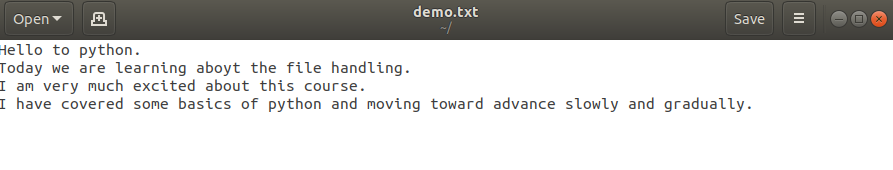## Polymorphism in python

##### Checkout the Detailed Explanation of Polymorphism with Examples
Written by |10-Apr-2019 | 0 Comments | 340 Views

Polymorphism is a technique by which we can change the attributes and normal functioning of the class. There are two type of polymorphism. Following are the names:

• Method over riding

Method overriding:

Method overrides refer to making same name method as defined in the parent class and changing the functionality of function. Sometimes we have to alter the functions functionality that is given in a base class by default. OOP allow us to change the functionality by overriding the function. Consider the first example which is the normal class as we have studied earlier.

 `class MyClass(object):` `    def __init__(self):` `        self.value = 77`   `    def printValue(self):` `        print(f"{self.value} from MyClass")`   `class ChildClass(MyClass):` `    def __init__(self):` `        super().__init__()` `  ` `x = ChildClass()` `x.printValue()`   #Output 77 from MyClass

In the above example when we create an object and try to get the value it will return 77 as default. But what happen when we want to get the square value without making changes in the base class. Here comes the concept method overriding. The above example is converted into polymorphism to apply overriding function.

 `class MyClass(object):` `    def __init__(self):` `        self.value = 77`   `    def printValue(self):` `        print(f"{self.value} from MyClass")`   `class ChildClass(MyClass):` `    def __init__(self):` `        super().__init__()` `    def printValue(self):` `        self.value = self.value ** 2` `        print(f"{self.value} from ChildClass")` `x = ChildClass()` `x.printValue()`   #Output 5929 from ChildClass

Unlike in C++ and java we cannot define multiple function of the same in python method overloading. We have to do in a single function by using default values. These values be than executed if there are no such values given by the user.

 `class MyClass(object):` `    def hello(self,name = "Anonymous"):` `        self.name = name` `        print (f"Hello {self.name}")`   `obj = MyClass()` `obj.hello()` `obj.hello('Ash Ketchum')`   #Output Hello Anonymous Hello Ash Ketchum

Similarly we can make parameterized and non-parameterized constructor  by the method over loading.

Now the classes are end here. Do practice the classes and make your own program with the help of these helping code.

File Handling:

File handling is the most important part for storing the data locally. Everytime we cannot make a database on sql or other services to store our data. Some time we store it locally in files. File handling is easy and fastest way to store the data and fetching the data. File handling have four important functions which are as follows:

• “w” : It writes the file and create the file if not present. Moreover, it will delete all the previous data if the file is present and write the new data.
• “a”: It append the data in already created file and not format the file as in case of file writing. It will generate an error if no such file found.
• “x” : It creates the file and return error if already exists.
• “t” : along with other function it will return text mode.
• “b”: along with other function it will return binary mode

File Writing:

File writing is a process to write a file and add data in it. Following is the example of writing the file:

 `text='''Hello to python.` `Today we are learning about the file handling.` `I am very much excited about this course.` `I have covered some basics of python and moving toward advance slowly and gradually.` `'''`   `filename = "demo.txt"` `file = open(filename,"wt")` `file.write(text)`   `file.close()`

After running this program, the text data is store in demo.txt file as shown in the image below:We can also read the file from the interpreter by following method:

 `filename = "demo.txt"` `file = open(filename,"rt")` `print(file.read())`   `file.close()`

 #output: Hello to python. Today we are learning aboyt the file handling. I am very much excited about this course. I have covered some basics of python and moving toward advance slowly and gradually.

readline() method returns all the line in a list which can be iterable. Following is the program to readlines:

 `filename = "demo.txt"` `file = open(filename,"rt")` `line = (file.readlines())` `print (line)` `for l in line:` `    print(l)` `file.close()`

Appending Data in a File:

We can append the data in already presented text file. Following is the example to add more data.

 `new_text='''Its a new text.` `I am adding some more lines.` `These line dont make any sense.` `Pikachu I choose you.'''`   `filename = "demo.txt"` `file = open(filename,"at")` `file.write(new_text)` `file.close`

Now we will see that if data is inserted or not. For this we must read the file and see the data. The output after reading the file we get:

 Hello to python. Today we are learning about the file handling. I am very much excited about this course. I have covered some basics of python and moving toward advance slowly and gradually. It’s a new text. I am adding some more lines. These lines don’t make any sense. Pikachu I choose you.

CSV writing:

CSV file are also called as comma separated file are used as alternative of excel sheets. It is extensively used in the process of data analysis. It is used to store data. Following is read and write operation on csv file:

Write CSV:

 `import csv`   `data = [['id','name'],['1','Pikachu'],['2','Blazikane']]`   `with open('pokemon.csv','w') as csv_file:` `    writer = csv.writer(csv_file)` `    writer.writerows(data)` `csv_file.close()`

 `import csv`   `with open('pokemon.csv','r') as csv_file:` `    reader = csv.reader(csv_file)` `    print(list(reader))` `csv_file.close()`

The output of the following code is :

`[['id', 'name'], ['1', 'Pikachu'], ['2', 'Blazikane']]`

With the help of csv file we can do a lot of things it is veru easy to handle csv file thanf txt file when it comes to data analysis and maintaining it. We will do advance work on CSV in out data analysis portion.